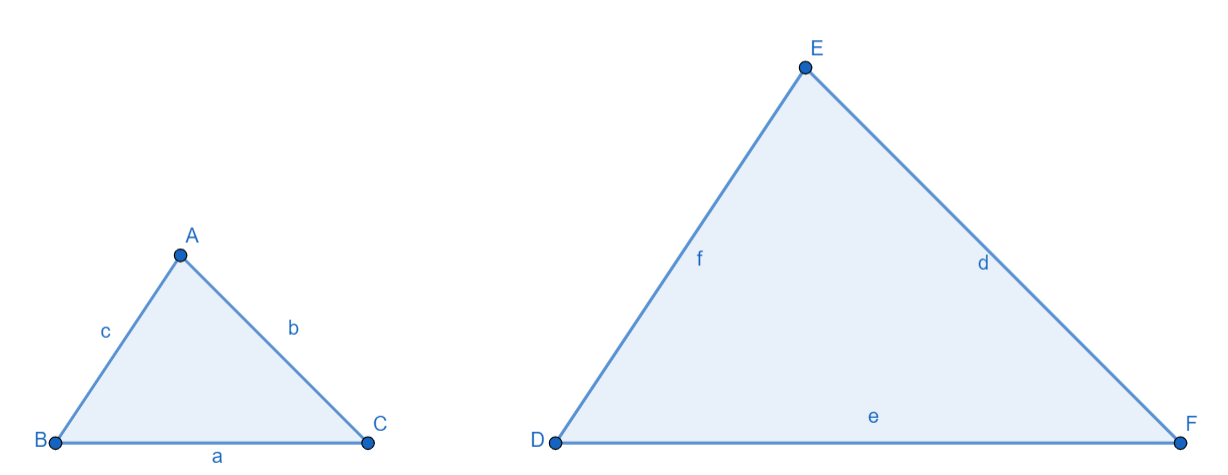Courses
Courses for Kids
Free study material
Free LIVE classes
MoreLIVE
Join Vedantu’s FREE Mastercalss

# Fill in the blanks using the correct word given in the brackets: All __________ triangles are similar. (Isosceles, Equilateral)Verified
363k+ views
Hint: The question is related to the similarity of triangles. Try to recall the AAA criterion for similarity of triangles and use the property that all the internal angles of an equilateral triangle measure ${{60}^{o}}$.The two triangles $\Delta ABC$ and $\Delta EDF$ are similar if $\dfrac{a}{e}=\dfrac{b}{d}=\dfrac{c}{f}$ . Also, if the two triangles are similar then, $\measuredangle A=\measuredangle E;\measuredangle B=\measuredangle D$ and $\measuredangle C=\measuredangle F$.
In case of an equilateral triangle, the three angles of the triangle are equal and their measure is fixed, i.e. ${{60}^{o}}$ . So, in all equilateral triangles, the measure of the angles is fixed and hence, the angles of two equilateral triangles will be equal. So, two equilateral triangles will always be similar.